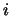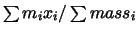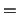Next: Analysis scripts Up: Molecular Analysis Previous: Using the molinfo command   Contents   Index

Using the atomselect command

Atom selection is the primary method to access information about the atoms in a molecule. It works in two steps. The first step is to create a selection given the selection text, molecule id, and optional frame number. This is done by a function called atomselect, which returns the name of the new atom selection. the second step is to use the created selection to access the information about the atoms in the selections.

Atom selection is implemented as a Tcl function. The data returned from atomselect is the name of the function to use. The name is of the form atomselect%d where '%d' is a non-negative number (such as 'atomselect0', atomselect26', ...).

The way to use the function created by the atomselect command is to store the name into a variable, then use the variable to get the name when needed.

vmd> set sel [atomselect top "water"]
atomselect3
vmd> $sel text water This is equivalent to saying vmd> atomselect3 text The easiest way of thinking about this is that the atomselect command creates an object. To get information from the object you have to send it a command. Thus, in the example above (atomselect1 num) the object "atomselect1" was sent the command "num", which asks the object to return the number of atoms in the selection. These derived object functions (the ones with names like atomselect3) take many options, as described in section §, For instance, given the selection vmd> set sel [atomselect top "resid 4"] atomselect4 you can get the atom names for each of the atoms in the selection with vmd>$sel get name
{N} {H} {CA} {CB} {C} {O}
(which, remember, is the same as
vmd> atomselect4 get name
)

Multiple attributes can be requested by submitting a list, so if you want to see which atoms are on the backbone,

vmd> $sel get {name backbone} {N 1} {H 0} {CA 1} {CB 0} {C 1} {O 1} and the atom coordinates with vmd>$sel get {x y z}
{0.710000 4.211000 1.093000} {-0.026000 3.700000 0.697000} {0.541000
4.841000 2.388000} {-0.809000 4.462000 2.976000} {1.591000 4.371000
3.381000} {2.212000 5.167000 4.085000}

It is very important to note that the information returned by one of these commands is a list of lists. Specifically, it is a list of sizewhere each element is itself a list of size, whereis the number of atoms in the selection andis the number of attributes requested.

One quick function you can build with the coordinates is a method to calculate the geometrical center (not quite the center of mass; that's a bit harder). This also uses some of the vector commands discussed in the section about vectors and matrices, but you should be able to figure them out from context.

proc geom_center {selection} {
# set the geometrical center to 0
set gc [veczero]
# [$selection get {x y z}] returns a list of {x y z} # values (one per atoms) so get each term one by one foreach coord [$selection get {x y z}] {
# sum up the coordinates
set gc [vecadd $gc$coord]
}
# and scale by the inverse of the number of atoms
return [vecscale [expr 1.0 /[$selection num]]$gc]
}

With that defined you can say (assuming $sel was created with the previous atomselection example) vmd> geom_center$sel
0.703168 4.45868 2.43667
I'll go through the example line by line. The function is named geom_center and takes one parameter, the name of the selection. The first line sets the variable gc'' to the zero vector, which is 0 0 0. On the second line of code, two things occur. First, the command
$selection get {x y z} is executed, and the string is replaced with the result, which is {0.710000 4.211000 1.093000} {-0.026000 3.700000 0.697000} {0.541000 4.841000 2.388000} {-0.809000 4.462000 2.976000} {1.591000 4.371000 3.381000} {2.212000 5.167000 4.085000} This is a list of 6 terms (one for each atom in the selection), and each term is a list of three elements, the x, y, and z coordinate, in that order. The "foreach" command splits the list into its six terms and goes down the list term by term, setting the variable "coord" to each successive term. Inside the loop, the value of$coord is added to total sum.

The last line returns the geometrical center of the atoms in the selection. Since the geometrical center is defined as the sum of the coordinate vectors divided by the number of elements, and so far I have only calculated the sum of vectors, I need the inverse of the number of elements, which is done with the expression

expr 1.0 / [$selection num] The decimal in "1.0" is important since otherwise Tcl does integer division. Finally, this value is used to scale the sum of the coordinate vectors (with vecscale), which returns the new value, which is itself returned as the result of the procedure. The center of mass function is slightly harder because you have to get the mass as well as the x, y, z values, then break that up into to components. The formula for the center of mass isproc center_of_mass {selection} { # some error checking if {[$selection num] <= 0} {
error "center_of_mass: needs a selection with atoms"
}
# set the center of mass to 0
set com [veczero]
# set the total mass to 0
set mass 0
# [$selection get {x y z}] returns the coordinates {x y z} # [$selection get {mass}] returns the masses
# so the following says "for each pair of {coordinates} and masses,
#  do the computation ..."
foreach coord [$selection get {x y z}] m [$selection get mass] {
# sum of the masses
set mass [expr $mass +$m]
# sum up the product of mass and coordinate
set com [vecadd $com [vecscale$m $coord]] } # and scale by the inverse of the number of atoms if {$mass == 0} {
error "center_of_mass: total mass is zero"
}
# The "1.0" can't be "1", since otherwise integer division is done
return [vecscale [expr 1.0/$mass]$com]
}

vmd> center_of_mass $sel Info) 0.912778 4.61792 2.78021 The opposite of "get" is "set". A few of the keywords (most notably, "x", "y", and "z") can be set to new values. This allows, for instance, atom coordinates to be changed, the occupancy values to be updated, or user forces to be added. Right now the only things that can be changed are the coordinates, the beta and occupancy values and user forces. set sel [atomselect top "index 5"]$sel get {x y z}
{1.450000 0.000000 0.000000}
$set set {x y z} {{1.6 0 0}} Note that just as the get option returned a list of lists, the set option needs a list of lists, which is why the extra set of curly braces were need. Again, this must be a list of sizecontaining elements which are a list of size. The exeception is ifis 1, the list is duplicated enough times so there is one element for each atom. # get two atoms and set their coordinates set sel [atomselect top "index 6 7"]$sel set {x y z} { {5 0 0} {7.6 5.4 3.2} }

In this case, the atom with index 6 gets its (x, y, z) values set to 5 0 0 and the atom with index 7 has its coordinates changed to 7.6 5.4 3.2.

It is possible to move atoms this way by getting the coordinates, changing them (say by adding some offset) and replacing it. Following is a function which will do just that:

proc moveby {sel offset} {
set newcoords {}
foreach coord [$sel get {x y z}] { lvarpush newcoords [vecadd$coord $offset] }$sel set $newcoords } And to use this function (in this case, to apply an offset of (x y z)(0.1 -2.8 9) to the selection "$movesel"):
moveby $movesel {0.1 -2.8 9} However, to simplify matters some options have been added to the selection to deal with movements (these commands are also implemented in C++ and are much faster than the Tcl versions). These functions are moveby, moveto, and move. The first two take a position vector and the last takes a transformation matrix. The first command, moveby, moves each of the atoms in the selection over by the given vector offset.$sel moveby {1 -1 3.4}
The second, moveto, moves all the atoms in a selection to a given coordinate (it would be strange to use this for a selection of more than one atom, but that's allowed). Example:
$sel moveto {-1 1 4.3} The last of those, move, applies the given transformation matrix to each of the atom coordinates. This is best used for rotating a set of atoms around a given axis, as in$sel move [trans x by 90]
which rotates the selection 90 degrees about the x axis. Of course, any transformation matrix may be used.

A more useful example is the following, which rotates the side chain atoms around the CA-CB bond by 10 degrees.

# get the sidechain atoms (CB and onwards)
set sidechain [atomselect top "sidechain residue 22"]
# get the CA coordinates -- could do next two on one line ...
set CA [atomselect top "name CA and residue 22"]
set CAcoord [lindex [$CA get {x y z}] 0] # and get the CB coordinates set CB [atomselect top "name CB and residue 22"] set CBcoord [lindex [$CB get {x y z}] 0]
# apply a transform of 10 degrees about the given bond axis
$sidechain move [trans bond$CAcoord \$CBcoord 10 deg]Next: Analysis scripts Up: Molecular Analysis Previous: Using the molinfo command   Contents   Index
vmd@ks.uiuc.edu